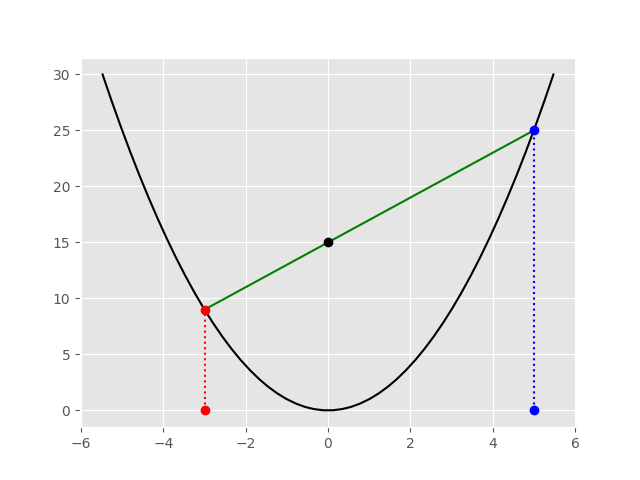# Multiplication via parabola

Here’s a graphical way to multiply two positive numbers a and b using the parabola y = x².

1. Start at the origin, move a units to the left, then go up vertically to the parabola, and draw a point.
2. Go back to the origin, move b units to the right, go up vertically to the parabola, and draw another point.
3. Connect the points and see where they cross the y-axis. That point is ab.

Here’s an example multiplying 3 and 5.Here’s why this works. The slope of the line is the change in y over the change in x which is

m = (b² − a²)/(b − (−a)) = ba.

Use the equation of a line

yy0 = m(xx0)

with x0 = b and y0 = b² to get

y − b² = (ba)(xb).

Stick in x = 0 and you get y = ab.

## 7 thoughts on “Multiplication via parabola”

1. Carlos Luna

There is a quite nice application of this property: the Parabolic Sieve of prime numbers!

2. I liked it! I will teach it to my students when they learn about parabolas.

3. @Michael: I think some mental calculators use that identity too.

4. Nice